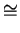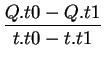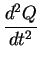Next:4.3.1.4 Model DefinitionUp:4.3.1 The Model DefinitionPrevious:4.3.1.2 Operators and Functions

### 4.3.1.3 Time Dependent Variables

Since AMI supports PDEs which might be time dependent every model has implicitly defined time dependent variables even if they are not used. Each quantity defined in the solution vector of an analytical model has got three different time-steps that represent the current state and the state of the last two time iterations. Furthermore, AMI predefines the variable t that holds the current calculated time (t0) and again the time of the two last calculated time-steps (tt - 1,tt - 2). The syntax used to distinguish between different times within the model description language looks like:
t   =  t.t0            # time-step t
t.t1            # time-step t-1
t.t2            # time-step t-2

<Q> = <Q.t0>           # value of the quantity Q at time t
<Q.t1>           # value of the quantity Q at time t-1
<Q.t2>           # value of the quantity Q at time t-2
With this mechanisms it is possible to handle discretized derivatives of time:
• first order discretization

•(4.2)
• second order discretization
•(4.3)Next:4.3.1.4 Model DefinitionUp:4.3.1 The Model DefinitionPrevious:4.3.1.2 Operators and Functions